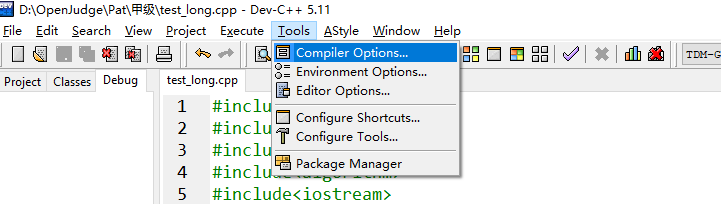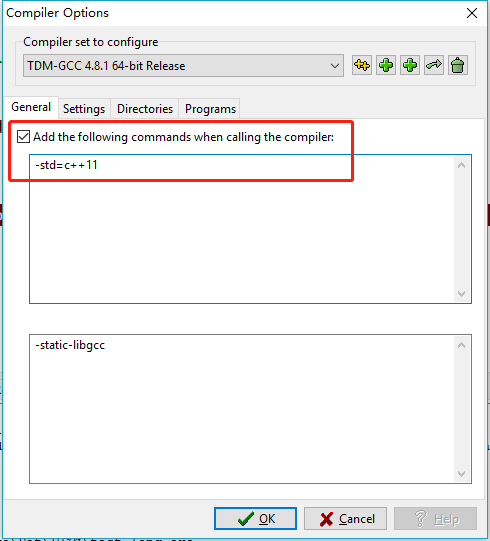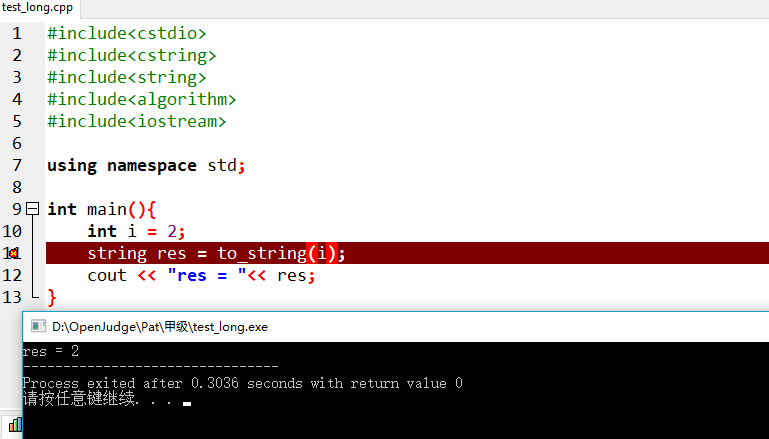c++5.11使用方法
2016-12-13 14:59:48 qq_36393555 阅读数 227
﻿﻿

```#include<iostream>
#include<string>

using namespace std;

int main()
{
string str;
int entercnt, tcnt, spacecnt;
entercnt = tcnt = spacecnt = 0;
while (getline(cin,str))
{
for (string::size_type i = 0; i < str.size(); ++i)
{
switch (str[i])
{
case'\n':++entercnt;
break;
case'\t':++tcnt;
break;
case' ':++spacecnt;
break;
}
}
}
cout << "换行有" << entercnt << "个" << endl
<< "制表符有" << tcnt << "个" << endl
<< "空格有" << spacecnt << "个" << endl;
return 0;
}

```

2018-01-01 00:41:49 swift2010 阅读数 769

Dev-Cpp 5.11 c++编译器下载地址：

2019-02-08 15:36:29 liu16659 阅读数 392

`Dev-C++ 5.11`不支持`C++ 11`编译

1.问题

Dev-C++ 不支持 C++ 11新特性。例如在使用编译如下这段代码时，就会报错。

``````#include<cstdio>
#include<cstring>
#include<string>
#include<algorithm>
#include<iostream>

using namespace std;

int main(){
int i = 2;
string res = to_string(i);
cout << "res = "<< res;
}
``````2. 修正办法

step 1：step 2：2014-07-30 10:29:20 jierandefeng 阅读数 923
﻿﻿

C++ primer(第五版） 练习 5.11

```#include <iostream>
#include <string>

using std::cout;
using std::cin;
using std::endl;
using std::string;
using std::getline;

int main()
{

unsigned aCnt = 0, eCnt = 0, iCnt = 0, oCnt = 0, uCnt = 0,spaceCnt=0,tCnt=0,nCnt=0;
string ch;

while (getline(cin,ch))
{
for (auto a : ch)
{
switch (a)
{
case 'a':
case 'A':
++aCnt;
break;
case 'e':
case 'E':
++eCnt;
break;
case 'i':
case 'I':
++iCnt;
break;
case 'o':
case 'O':
++oCnt;
break;
case 'u':
case 'U':
++uCnt;
break;
case ' ':
++spaceCnt;
break;
case '\t':
++tCnt;
break;
case '\n':
++nCnt;
break;

}
}
}

cout << "Number of vowel a or A: \t" << aCnt << '\n'
<< "Number of vowel e or E: \t" << eCnt << '\n'
<< "Number of vowel i or I: \t" << iCnt << '\n'
<< "Number of vowel o or O: \t" << oCnt << '\n'
<< "Number of vowel u or U: \t" << uCnt << '\n'
<< "Number of  space: \t" << spaceCnt << '\n'
<< "Number of \'\\t\': \t" << tCnt << '\n'
<< "Number of \'\\n\': \t" << nCnt << endl;

return 0;

}

```2017-05-11 23:11:09 Qin_xian_shen 阅读数 152

C++程序设计语言练习5.11 map标准库应用

C++标准库

Dev c++ 5.11 调试方法入门 初学者适用

Devc++虽然功能上和美观度上比不上CodeBlocks和sublime，但这个IDE调试还是比较简单的，在OI或ACM上用到的可能性还是有的。许多人的Dev有一调试就闪退GDB报error的情况本人开始也遇到了解决方案如下打开编译选项把产生调试信息打开（变成yes后面会有图片）之后在编写完代码后开始调试让我们随便写点直接在行标前点一...

Debug日志：Dev C++ 5.11的 int 型边界测试

int型变量在内存中占4个字节，这在以前已经用sizeof函数测试过。现在做更加细化的测试：#includeusingnamespacestd;intmain(){ cout

c++ 模版的使用方法

visual C++使用方法！！！！！！！！！！！！

http://tech.china.com/zh_cn/netschool/programme/c/656/20001207/vc01.html